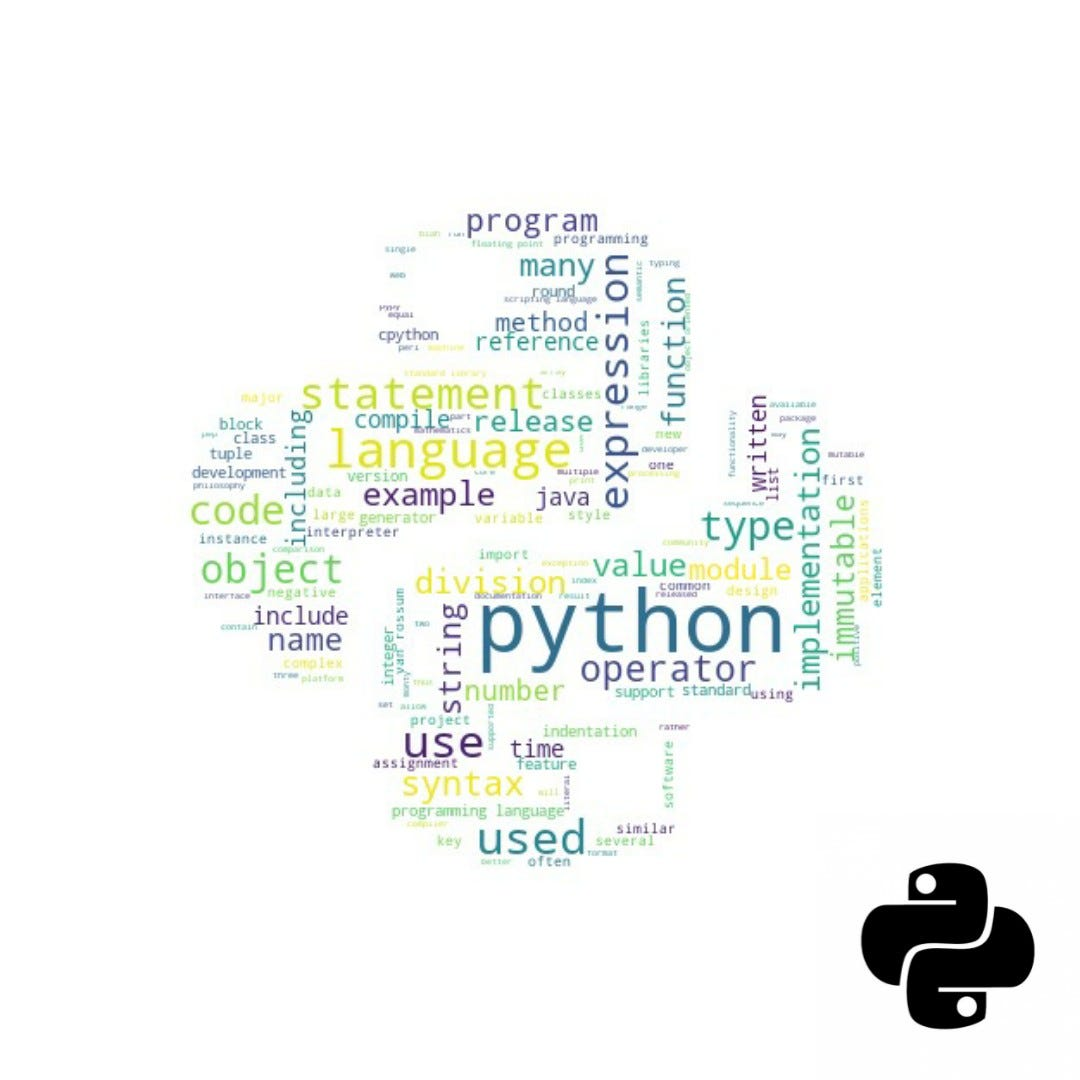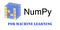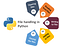# NumPy & File Handling

## Python’s beginner libraries to start Machine Learning.Credit: AuthorNumPy — a library of arrays
`#Note: Here, I used italic to show output.import numpy as nparr = np.array([1, 2, 3, 4, 5])print(arr)#[1 2 3 4 5]#Creating zeros matrixnp.zeros((2,3))#array([[0., 0., 0.],       [0., 0., 0.]])#Creating ones matrixnp.ones(5,dtype=np.int32)#array([1, 1, 1, 1, 1])#Creating matrix with random datanp.random.rand(2, 3)#array([[0.95580785, 0.98378873, 0.65133872],       [0.38330437, 0.16033608, 0.13826526]])#Flattening or changing shape of matrixa = np.ones((2,2))print('Original shape :', a.shape)print('Array :','\n', a)print('Shape after flatten :',b.shape)#Original shape : (2, 2)Array :  [[1. 1.] [1. 1.]]Shape after flatten : (4,)Array :  [1. 1. 1. 1.]#Stacking matrix both horizontally and vertically #Arange create a matrix from given rangea = np.arange(0,5)b = np.arange(5,10)print('Array 1 :','\n',a)print('Array 2 :','\n',b)print('Vertical stacking :','\n',np.vstack((a,b)))print('Horizontal stacking :','\n',np.hstack((a,b)))#Array 1 :  [0 1 2 3 4]Array 2 :  [5 6 7 8 9]Vertical stacking :  [[0 1 2 3 4] [5 6 7 8 9]]Horizontal stacking :  [0 1 2 3 4 5 6 7 8 9]#Type of data structure b = np.array([3.1, 11.02, 6.2, 213.2, 5.2])type(b)#numpy.ndarray#data-type of arrayb.dtype #dtype(‘float64’)# Slicing the numpy arrayd = b[1:4]print(d)#[ 11.02   6.2  213.2 ]# Get the number of dimensions of numpy arrayb.ndim           #1# Get the shape/size of numpy arrayb.shape         #(5,)# Get the mean of numpy arraymean = b.mean()    #47.74399999999999# Get the standard deviation of numpy arraystandard_deviation=b.std()          #82.76874134599365# Get the biggest value in the numpy arraymax_b = b.max()    #213.2# Get the smallest value in the numpy arraymin_b = b.min()      #3.1# MATRIX Multiplicationmat1 = ([1, 6, 5],[3 ,4, 8],[2, 12, 3])mat2 = ([3, 4, 6],[5, 6, 7],[6,56, 7])np.dot(mat1, mat2)#array([[ 63, 320,  83],       [ 77, 484, 102],       [ 84, 248, 117]])# Pi is math functionnp.pi3.141592653589793# Calculate the sin of each elementsy = np.sin(b)#array([ 0.04158066, -0.99970171, -0.0830894 , -0.41532536, -0.88345466])# Makeup a numpy array within [-2, 2] and 5 elementsnp.linspace(-2, 2, num=5)  #array([-2., -1.,  0.,  1.,  2.])`

NOTE : In Python, we read images as NumPY arrays, which you will see in further blogs of deep learning.Handling FILES via Python
`# OPENING FILE#1st Wayfileref = open("olympics.txt", "r") #fileref is reference (opening a file in python3)#opening file via relative path#open('/Users/joebob01/myFiles/allProjects/myData/data2.txt', 'r')#2nd way to open filewith open("olympics.txt","r") as fileref :#lines of code to work on file ....#automatically closes file#READING and processing a file :with open('fname', 'r') as fileref:         # step 1lines = fileref.readlines()           # step 2 - get list of all** lines of text in filefor lin in lines:              # step 3 - for loop to iterate lines#WRITING in a filewith open("filename","w") as f1 :for i in range(5) :sqr = i*if1.write(str(sqr) + "\n")   #also write data as int, float#NOTE : File open in write 'w' mode overwrite the content (i.e deletes past content and save only new content) whereas in append mode 'a' data in added to end of past content#CLOSING a file fileref.close()#closing file is necessary to prevent misuse by other users#DELETING fileimport osif os.path.exists("demofile.txt"):  os.remove("demofile.txt")else:  print("The file does not exist")`

Learning and exploring this beautiful world with amazing tech.

## More from Deep Patel

Learning and exploring this beautiful world with amazing tech.